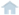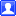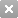码农库 > 编程语言和算法 > C/C++ > C++ 打印九九剩法表

C++ 打印九九剩法表发表于 10月2日 22:35

1楼发表于 10月2日 22:36

``````1 × 1 =  1

1 × 2 =  2  2 × 2 =  4

1 × 3 =  3  2 × 3 =  6  3 × 3 =  9

1 × 4 =  4  2 × 4 =  8  3 × 4 = 12  4 × 4 = 16

1 × 5 =  5  2 × 5 = 10  3 × 5 = 15  4 × 5 = 20  5 × 5 = 25

1 × 6 =  6  2 × 6 = 12  3 × 6 = 18  4 × 6 = 24  5 × 6 = 30  6 × 6 = 36

1 × 7 =  7  2 × 7 = 14  3 × 7 = 21  4 × 7 = 28  5 × 7 = 35  6 × 7 = 42  7 × 7 = 49

1 × 8 =  8  2 × 8 = 16  3 × 8 = 24  4 × 8 = 32  5 × 8 = 40  6 × 8 = 48  7 × 8 = 56  8 × 8 = 64

1 × 9 =  9  2 × 9 = 18  3 × 9 = 27  4 × 9 = 36  5 × 9 = 45  6 × 9 = 54  7 × 9 = 63  8 × 9 = 72  9 × 9 = 81``````

【本版规则】请勿发表违反国家法律的内容，否则会被冻结账号和删贴。
 用户名： 立即注册 密码：2020-2023 MaNongKu.com